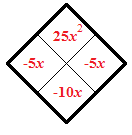### Home > CCA > Chapter 9 > Lesson 9.1.3 > Problem9-27

9-27.

Solve the following quadratic equations by factoring and using the Zero Product Property. Then check your solutions.

1. $x^2-10x+25=0$

Use a generic rectangle and a diamond problem to help you factor the equation.

 left edge top $-5$ interior top left $-5x$ interior top right $25$ left edge bottom$x$ interior bottom left $x^2$ interior bottom right $-5x$ bottom edge left$x$ bottom edge right$-5$$x=5$

1. $0=3x^2+17x-6$

See part (a).

1. $3x^2-2x=5$

Rewrite the equation so that it is equal to $0$.

$x=\frac{5}{3},\ x=-1$

1. $16x^2-9=0$

You should notice that this is a difference of squares.